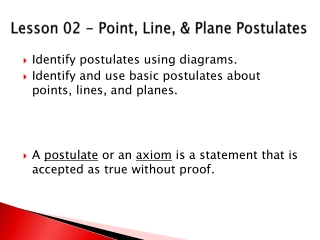Download PresentationLesson 02 - Point, Line, & Plane Postulates

# Lesson 02 - Point, Line, & Plane Postulates

Télécharger la présentation## Lesson 02 - Point, Line, & Plane Postulates

- - - - - - - - - - - - - - - - - - - - - - - - - - - E N D - - - - - - - - - - - - - - - - - - - - - - - - - - -
##### Presentation Transcript

1. Lesson 02 - Point, Line, & Plane Postulates • Identify postulates using diagrams. • Identify and use basic postulates about points, lines, and planes. • A postulate or an axiom is a statement that is accepted as true without proof.

2. Two Point Postulate (Card #1) Through any two points there exists exactly one line.

3. Line-Point Postulate(Card #2) A line contains at least two points.

4. Line Intersection Postulate (Card #3) If two lines intersect, then their intersection is exactly one point.

5. Three Point Postulate (Card #4) Through any three non-collinear points, there exists exactly one plane.

6. Plane Point Postulate (Card #5) A plane contains at least three non-collinear points.

7. Plane Line Postulate (Card #6) If two points lie in a plane, then the line containing them lies in the plane.

8. Plane Intersection Postulate (Card #7) If two planes intersect, then their intersection is a line.